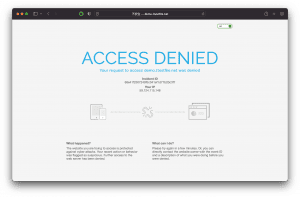Home » Blog » How Mlytics patched Cloudflare WAF bypass vulnerability (on our end)

# How Mlytics patched Cloudflare WAF bypass vulnerability (on our end)On Oct 25, 2018, a researcher from ODS (Open Data Security) named Daniel Fariña released a blog post sharing his findings about a vulnerability in the case of Nginx on Cloudflare, which could disable the WAF leaving the companies vulnerable to cyber attacks. There’s even a video providing a very detailed explanation and demonstration of this issue.

This finding has caught our attention as we are also using Nginx to develop our own WAF (see article: Why and How Mlytics Built Its Own Web Application Firewall).

## What happened exactly?

We noticed that Lua in Nginx has a limitation in terms of accessibility to all the information of one request, and it can be summarized as follow :

“… a maximum of 100 request arguments are parsed by default (including those with the same name) and that additional request arguments are silently discarded to guard against potential denial of service attacks”.

Meaning which, any WAF developed on top of Nginx in this scenario will be left vulnerable if one request is using this as a loophole to keep everything undetected. If the parameters that contain threats are not being supported within the scope, it will be totally unusable.

## So why do we have to patch anything?

At Mlytics, we give our users the liberty to choose what CDN platforms to use, while keeping them protected via our proprietary WAF to safeguard and unify security policy across platforms. Since some of our users have enabled Cloudflare via Mlytics’ Multi CDN, thus making them vulnerable to this issue.

We didn’t wait long for Cloudflare to patch the hole. We can intercept malicious requests that bypass Cloudflare’s Lua-Nginx vulnerability via a patch to the Mlytics platform to keep our users protected.

## It works!

We did a couple of before and after tests, here are the results:

Before patch
Test scenario: with one parameter

“`curl -i ‘127.0.0.1/?txtSearch=<%21–%23cmd’ -H “Host: demo.1testfire.net”
HTTP/1.1 403 Forbidden
Server: nginx
Date: Thu, 13 Dec 2018 07:08:05 GMT
Content-Type: text/html
Transfer-Encoding: chunked
Connection: keep-alive
Cache-Control: no-cache

Incident ID 68e41f2507249f5c041ef1d77b20c3f7What happened ?The website you are trying to access is protected against cyber attacks. Your recent action or behavior was flagged as suspicious. Further access to the web server has been denied.

What can I do ?Please try again in a few minutes. Or, you can directly contact the site owner within Event ID indicated and a description of what you were doing before you were denied access.

“`

Test scenario: with a0-a9, 10*10, a total of 100 parameters, and then add the 101st parameters to the command injection payload

“`curl -i ‘127.0.0.1/?a0=0&a0=0&a0=0&a0=0&a0=0&a0=0&a0=0&a0=0&a0=0&a0=0&a1=1&a1=1&a1=1&a1=1&a1=1&a1=1&a1=1&a1=1&a1=1&a1=1&a2=2&a2=2&a2=2&a2=2&a2=2&a2=2&a2=2&a2=2&a2=2&a2=2&a3=3&a3=3&a3=3&a3=3&a3=3&a3=3&a3=3&a3=3&a3=3&a3=3&a4=4&a4=4&a4=4&a4=4&a4=4&a4=4&a4=4&a4=4&a4=4&a4=4&a5=5&a5=5&a5=5&a5=5&a5=5&a5=5&a5=5&a5=5&a5=5&a5=5&a6=6&a6=6&a6=6&a6=6&a6=6&a6=6&a6=6&a6=6&a6=6&a6=6&a7=7&a7=7&a7=7&a7=7&a7=7&a7=7&a7=7&a7=7&a7=7&a7=7&a8=8&a8=8&a8=8&a8=8&a8=8&a8=8&a8=8&a8=8&a8=8&a8=8&a9=9&a9=9&a9=9&a9=9&a9=9&a9=9&a9=9&a9=9&a9=9&a9=9&<%21–%23cmd’ -H “Host: demo.1testfire.net”
HTTP/1.1 200 OK
Server: nginx
Date: Thu, 13 Dec 2018 07:20:29 GMT
Content-Type: text/html; charset=utf-8
Transfer-Encoding: chunked
Connection: keep-alive
Cache-Control: no-cache
Pragma: no-cache
Expires: -1
X-AspNet-Version: 2.0.50727
X-Powered-By: ASP.NET
http://www.w3.org/TR/xhtml1/DTD/xhtml1-transitional.dtd”>
http://www.w3.org/1999/xhtml” xml:lang=”en” >

As you can tell before our patch, a request within 100 parameters can be easily blocked by the Cloudflare WAF. But once it goes above 100 and hit its 101st parameters, Cloudflare WAF became inactive and let it pass.

After patch
Test scenario: with one parameter

“`curl -i ‘127.0.0.1/?txtSearch=<%21–%23cmd’ -H “Host: demo.1testfire.net”
HTTP/1.1 403 Forbidden
Server: nginx
Date: Thu, 13 Dec 2018 07:08:05 GMT
Content-Type: text/html
Transfer-Encoding: chunked
Connection: keep-alive
Cache-Control: no-cache

Incident ID 68e41f2507249f5c041ef1d77b20c3f7What happened ?The website you are trying to access is protected against cyber attacks. Your recent action or behavior was flagged as suspicious. Further access to the web server has been denied.

What can I do ?Please try again in a few minutes. Or, you can directly contact the site owner within Event ID indicated and a description of what you were doing before you were denied access.

“`

Test scenario: with a0-a9, 10*10, a total of 100 parameters, and then add the 101st parameters to the command injection Payload

“`curl -i ‘127.0.0.1/?a0=0&a0=0&a0=0&a0=0&a0=0&a0=0&a0=0&a0=0&a0=0&a0=0&a1=1&a1=1&a1=1&a1=1&a1=1&a1=1&a1=1&a1=1&a1=1&a1=1&a2=2&a2=2&a2=2&a2=2&a2=2&a2=2&a2=2&a2=2&a2=2&a2=2&a3=3&a3=3&a3=3&a3=3&a3=3&a3=3&a3=3&a3=3&a3=3&a3=3&a4=4&a4=4&a4=4&a4=4&a4=4&a4=4&a4=4&a4=4&a4=4&a4=4&a5=5&a5=5&a5=5&a5=5&a5=5&a5=5&a5=5&a5=5&a5=5&a5=5&a6=6&a6=6&a6=6&a6=6&a6=6&a6=6&a6=6&a6=6&a6=6&a6=6&a7=7&a7=7&a7=7&a7=7&a7=7&a7=7&a7=7&a7=7&a7=7&a7=7&a8=8&a8=8&a8=8&a8=8&a8=8&a8=8&a8=8&a8=8&a8=8&a8=8&a9=9&a9=9&a9=9&a9=9&a9=9&a9=9&a9=9&a9=9&a9=9&a9=9&<%21–%23cmd’ -H “Host: demo.1testfire.net”
HTTP/1.1 403 Forbidden
Server: nginx
Date: Thu, 13 Dec 2018 07:18:51 GMT
Content-Type: text/html
Transfer-Encoding: chunked
Connection: keep-alive
Cache-Control: no-cache

Incident ID 68e41f2507249f5c041ef1d77b20c3f7